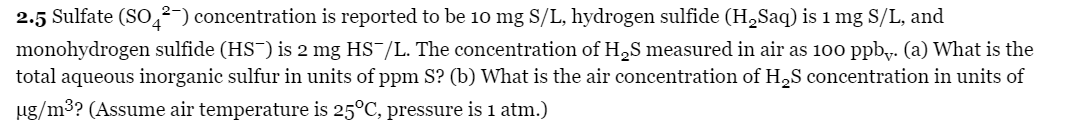Home / Expert Answers / Chemical Engineering / 2-5-sulfate-left-mathrm-so-4-2-right-concentration-is-reported-to-be-10-mathrm-pa292

# (Solved): 2.5 Sulfate $$\left(\mathrm{SO}_{4}^{2-}\right)$$ concentration is reported to be $$10 \mathrm{ ...2.5 Sulfate \( \left(\mathrm{SO}_{4}^{2-}\right)$$ concentration is reported to be $$10 \mathrm{mg} \mathrm{S} / \mathrm{L}$$, hydrogen sulfide $$\left(\mathrm{H}_{2} \mathrm{Saq}\right)$$ is $$1 \mathrm{mg} \mathrm{S} / \mathrm{L}$$, and monohydrogen sulfide $$\left(\mathrm{HS}^{-}\right)$$is $$2 \mathrm{mg} \mathrm{HS}^{-} / \mathrm{L}$$. The concentration of $$\mathrm{H}_{2} \mathrm{~S}$$ measured in air as $$100 \mathrm{ppb}_{\mathrm{v}}$$. (a) What is the total aqueous inorganic sulfur in units of $$\mathrm{ppm} \mathrm{S}$$ ? (b) What is the air concentration of $$\mathrm{H}_{2} \mathrm{~S}$$ concentration in units of $$\mu \mathrm{g} / \mathrm{m}^{3}$$ ? (Assume air temperature is $$25^{\circ} \mathrm{C}$$, pressure is $$1 \mathrm{~atm} .$$ )

We have an Answer from Expert